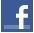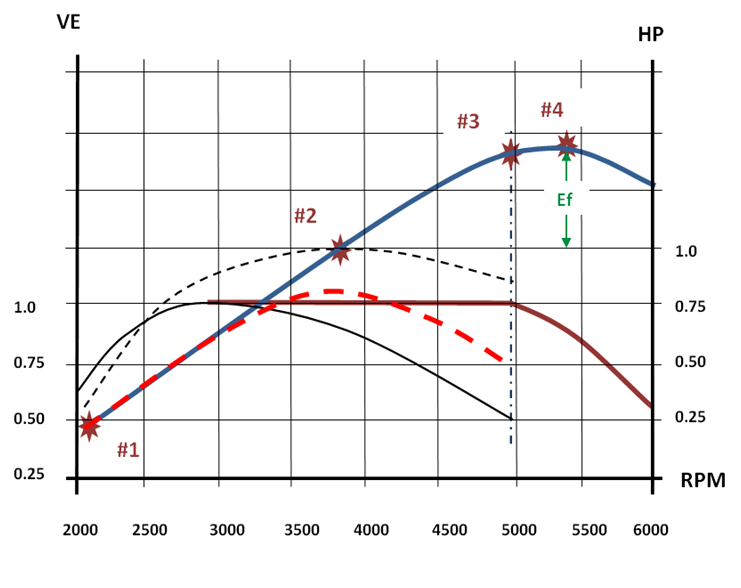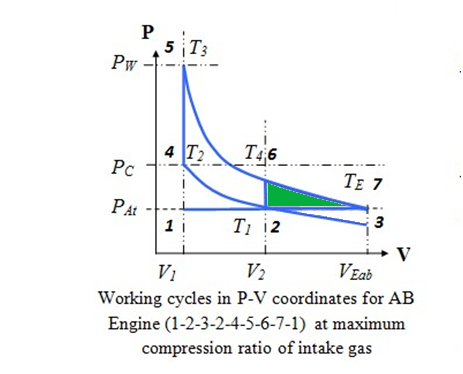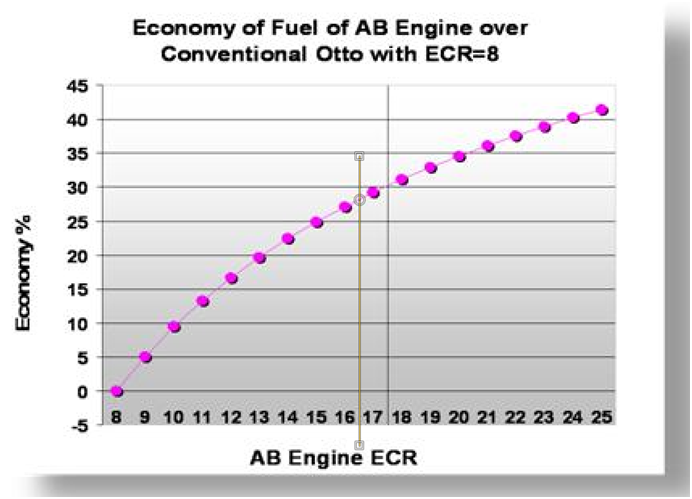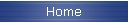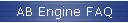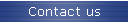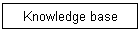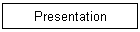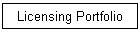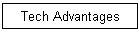AB Engine Fuel Efficiency

Calculation of efficiency of AB Engine and its comparison with the conventional engine.

Lets fist derive the expression for conventional engine, which is based on a standard Otto cycle.

Using notations in Fig.1 for conventional Otto cycle, the efficiency η can be expressed as follows:(1)

The fact that compression and expansion ratios are equal in conventional engine leads to the following relation: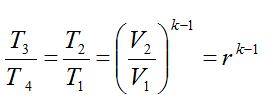(2)

Where r is a compression ratio for conventional Otto cycle, k is an effective value of γ from formula (3) which is equal to 1.35 for typical air-fuel mixture.

From (2) it follows that: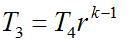(3)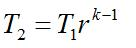(4)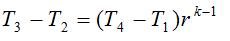(5)

From (1) and (5) we have: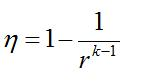(6)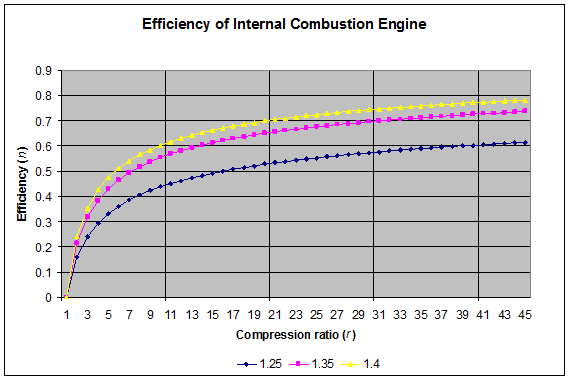Picture 1. Efficiency of conventional Internal Combustion Engine

The above is a classical derivation based on the proportional relation between change in internal energy and change in temperature and can be found in numerous classical textbooks as well as in recent publications. The one given here was taken from the following reference: http://www.engr.colostate.edu/~allan/thermo/page5/page5.htmlPicture 2. PV Diagram of the AB Engine 1-2-3-2-4-5-6-7-8-7 and

conventional engine 1-2-4-5-6-2 at maximum ICE cycle power

For the purpose of efficiency calculation in the proposed engine, it must be taken into consideration that the compression and expansion ratios are different. According to Picture 2, the gas is compressed above atmospheric pressure from volume V2 to V1 (trace 2-4), but expands from volume V1 to effective volume VE (trace 5-6-7). To account for this change the formula (1) must be modified as follows: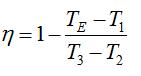(7)

The relation between temperatures and volumes during compression gives: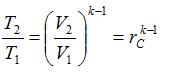(8)

where rC is the compression ratio for the proposed engine.

The similar relation for expansion gives: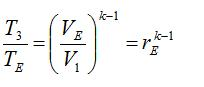(9)

where rE is the expansion ratio for the proposed engine.

Expressing T2 and TE from (7) and (8) and inserting them into (7) gives the following expression for η: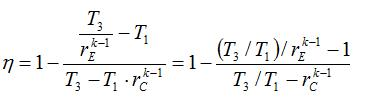(10)

Note that η now depends on the ratio T3 /T1, which has approximate value of 8 for most of typical gasoline engines. Assuming the compression ratio of best gasoline engines rC≈10 and twice higher expansion ratio of the proposed engine rE≈20, the value of η can be calculated as: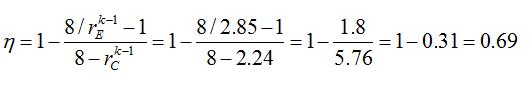(11)

The same formula (10) can be used to calculate η for conventional engine, which gives: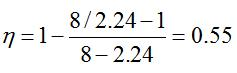(12)

Note that this value is in a perfect agreement with a classical formula (6):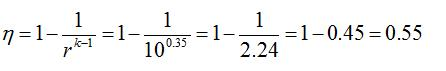(13)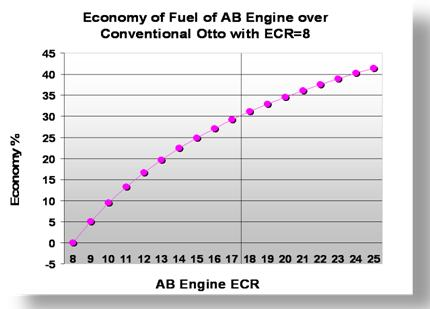Picture 3. The graph above shows how much an AB Engine can improve

fuel efficiency in comparison to a conventional engine with compression ratio r = 8

Visits since June 2, 2016:Tweet